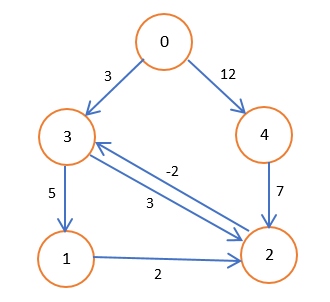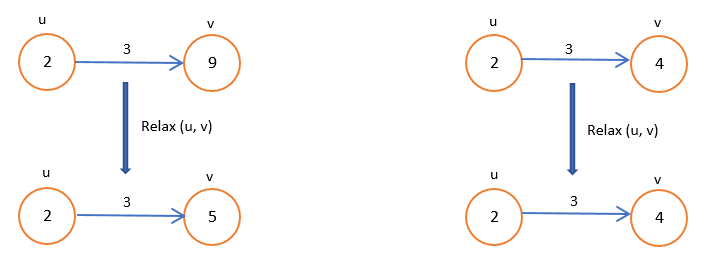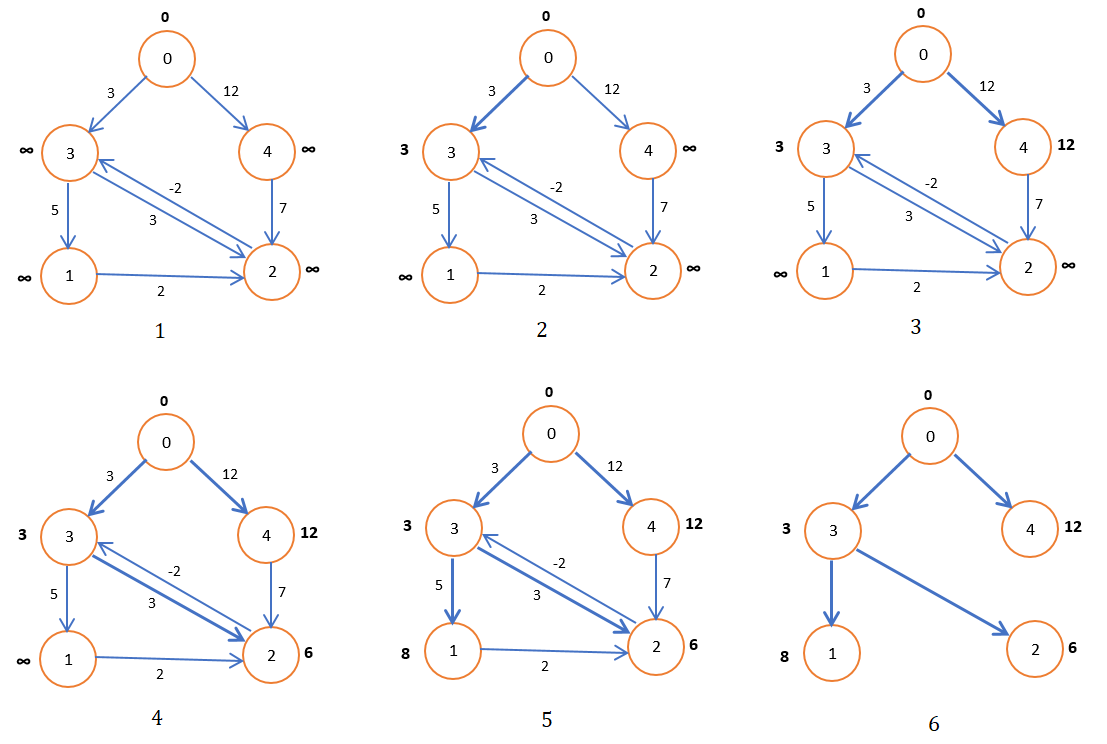# What is shortest path and implementation of Bellman-Ford Algorithm

 Category: Data Structure And Algorithms Tags:Bellman Ford Algorithm Code Files

## Introduction

Suppose you want to travel from one city to another then first you must see what is optimal route from set of routes so you can choose which has least distance/time. We call this route shortest path. To find shortest path we are given a directed graph G = {V, E}, a source “u” and a destination “v” vertex.

We can find single source shortest path to all destinations where we are given only source and we have to find shortest path to all destinations.

Weights, Negative Weights and Cycles

Weight is a value between two vertices or we can say value of an edge. However, we do not see negative weights in real-time scenarios but a graph can be given with negative weighted edges. If a graph has negative values still we can say it is well defined but if a graph has negative weighted cycle between vertex x and y then it can be said that there is no path between x and y so w(x, y) = -∞.

Whether a graph has positive or negative cycle we must remove all cycles to ease the calculation of shortest path.Fig 1: Directed Graph with Cycle

## Implementation

We will create classes for vertex and edge like below

```public class Vertex
{
public string Name { get; set; }
public int D { get; set; }
public Vertex Parent { get; set; }
}public class Edge
{
public int U { get; set; }
public int V { get; set; }
public int Weight { get; set; }
}```

Now we have to initialize all vertices with infinite weight and source with zero

```public static void InitializeSingleSource(Vertex[] vertices, Vertex s)
{
foreach (Vertex v in vertices)
{
v.D = int.MaxValue;
v.Parent = null;
}
s.D = 0;
}```

We have to write a method called Relax which sets minimum weight to a vertex and assigns parent to it

```public static void Relax(Vertex u, Vertex v, int weight)
{
if (v.D > u.D + weight)
{
v.D = u.D + weight;
v.Parent = u;
}
}```

Relax compares a destination weight with addition of weight of source vertex and weight of weight and sets which is smaller, given in figure below:Fig 2: Relaxation in bellman-ford algorithm

Now let’s implement Bellman-Ford’s algorithm

```public static bool BellmanFord(Vertex[] vertices, List<Edge> edges, int source)
{
//Initializing vertices
InitializeSingleSource(vertices, vertices[source]);

//Relaxing each edge by traversing from each vertex and so complexity is O(V.E)
for (int i = 0; i < vertices.Length - 1; i++)
{
foreach (Edge edge in edges)
{
Relax(vertices[edge.U], vertices[edge.V], edge.Weight);
}
}
//Checking if path exist or not to all vertices from source
foreach (Edge edge in edges)
{
Vertex u = vertices[edge.U];
Vertex v = vertices[edge.V];

if (v.D > u.D + edge.Weight)
{
return false;
}
}
return true;
}```

Algorithm returns true if there is path from source to all vertices else false. Now let's write method which prints path between two vertices

```public static void PrintPath(Vertex u, Vertex v, Vertex[] vertices)
{
if (v != u)
{
PrintPath(u, v.Parent, vertices);
Console.WriteLine("Vertax {0} weight: {1}", v.Name, v.D);
}
else
Console.WriteLine("Vertax {0} weight: {1}", v.Name, v.D);
}```

Now let’s print the path and weights between vertex 0 and 2:

```public static void Main(string[] args)
{
int[][] adjacencyMatrix = new int[][] { new int[] { 0,0,0,3,12 },
new int[] { 0,0,2,0,0 },
new int[] { 0,0,0,-2,0 },
new int[] { 0,5,3,0,0 },
new int[] { 0,0,7,0,0 } };

Vertex[] vertices = new Vertex[adjacencyMatrix.GetLength(0)];
List<Edge> edges = new List<Edge>();
//Source vertex
int source = 0;

for (int i = 0; i < vertices.Length; i++)
vertices[i] = new Vertex() { Name = i.ToString() };

//Insering all edges in list, treversing from source
Queue<int> verticesQueue = new Queue<int>();
verticesQueue.Enqueue(source);
while (verticesQueue.Count > 0)
{
int u = verticesQueue.Dequeue();
for (int v = 0; v < adjacencyMatrix.GetLength(0); v++)
{
if (adjacencyMatrix[u][v] > 0)
{
edges.Add(new Edge() { U = u, V = v, Weight = adjacencyMatrix[u][v] });
verticesQueue.Enqueue(v);
}
}
}
//Calling Bellman-Ford Algorithm
BellmanFord(vertices, edges, source);
//Prints path
PrintPath(vertices, vertices, vertices);

Console.ReadLine();
}```

Output

Vertax 0 weight: 0

Vertax 3 weight: 3

Vertax 2 weight: 6

Algorithm creates shortest path as shown in below figure:Fig 3: Bellman Ford Algorithm for Shortest Path

Algorithm finds shortest path of all routes from source and runs on O (V.E) time complexity.

 Like0 People
Last modified on 7 March 2019Nikhil Joshi Ceo & Founder at Dotnetlovers Atricles: 144 Questions: 15 Given Best Solutions: 15 *

## Comments:

No Comments Yet

You are not loggedin, please login or signup to add comments:

## Existing User

Login via:## New Userx